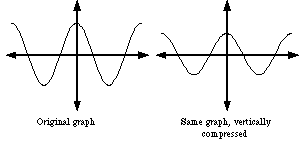index: click on a letter A B C D E F G H I J K L M N O P Q R S T U V W X Y Z A to Z index index: subject areas numbers & symbols sets, logic, proofs geometry algebra trigonometry advanced algebra & pre-calculus calculus advanced topics probability & statistics real world applications multimedia entrieswww.mathwords.com about mathwords website feedback

 Shrink Compression of a Graph A transformation in which all distances on the coordinate plane are shortened by multiplying either all x-coordinates (horizontal compression) or all y-coordinates (vertical compression) of a graph by a common factor less than 1. Note: When the common factor is greater than 1 the transformation is called a dilation or a stretch.See also Graph of an equation, compression of a geometric figure